# Regular and Irregular Polygons

In geometry, regular and irregular polygons are also called regular and irregular shapes. These are the types of polygons that differ from each other with respect to their dimensions. The polygon is a two-dimensional closed shape with all its sides connected to each other. The point at which two sides meet is called the vertex. The sides of the polygon are also called edges.

While understanding quadrilaterals, we have come across different types such as square, rectangle, rhombus, trapezium, parallelogram and kite. All these types of quadrilaterals are regular and irregular polygons, based on their respective properties.

In this article, we are going to learn the definition and types of regular and irregular polygons, along with their examples.

## Regular polygons

A regular polygon has all its sides equal and all its angles equal in measure. Examples of a regular polygon are square, equilateral triangle, rhombus, etc.

Let us see examples of regular polygons with figures.

### Equilateral Triangle

An equilateral triangle is a regular polygon that has all its sides equal to each other and the measure of all the angles is equal to 60 degrees. Therefore, it is also called a regular triangle. See the figure below.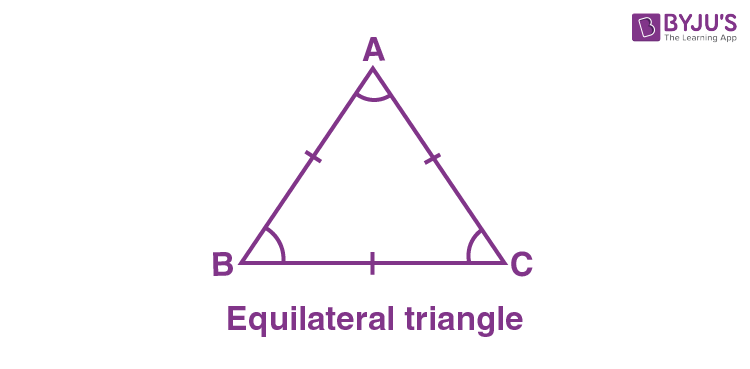In the above triangle ABC, the sides AB, BC and AC are equal to each other.

AB = BC = AC

Also, the angles are equal in measure.

∠A = ∠B = ∠C

How are all angles equal to 60°?

By angle sum property of triangle, we know that,

∠A + ∠B + ∠C = 180°

3∠A = 180°

∠A = 180/3 = 60°

Therefore, ∠A, ∠B and ∠C are all equal to 60°.

### Square

A square is a regular polygon that has all its sides equal in length and all its angles equal in measure. The angles of the square are equal to 90 degrees. See the figure below.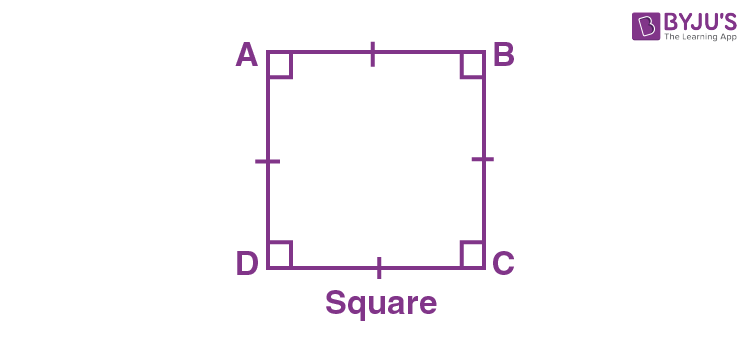In the square ABCD above, the sides AB, BC, CD and AD are equal in length.

AB = BC = CD = AD

Also, all the angles are equal in measure to 90 degrees.

∠A = ∠B = ∠C = ∠D = 90°

How are all the angles equal to 90°?

By the angle sum property of quadrilaterals, we now know that all the angles of a quadrilateral sum up to 360°.

∠A + ∠B + ∠C + ∠D = 360°

Since all the angles of a square are equal, therefore, we can replace all the angles with one angle.

∠A + ∠A + ∠A + ∠A = 360°

4∠A = 360°

∠A = 90°

Therefore, all the angles are also equal to 90°.

### Regular Pentagon

A regular pentagon is a two-dimensional closed shape that has all its five sides equal in length and all the five angles are equal in measure. Each interior angle of the regular pentagon is equal to 108°. See the figure below.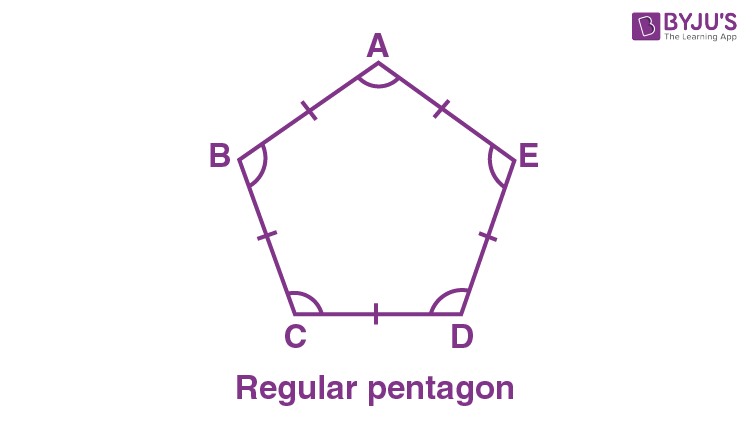In the above pentagon, the five sides AB, BC, CD, DE and AE are equal in length.

AB = BC = CD = DE = AE

All the angles are also equal.

∠A = ∠B = ∠C = ∠D = ∠E = 108°

The Sum of all the angles of a pentagon is equal to 540°. We can use the angle sum property of pentagons here to find how each angle is equal to 108°.

 Sum of all the interior angles of n-sided polygon = (n – 2) × 180°

### Regular Hexagon

A regular hexagon has six equal sides and six equal angles. Each interior angle of the regular hexagon is equal to 120°.

 A regular polygon has: All sides equal All angles equal

## Irregular polygons

An irregular polygon does not have all its sides equal and not all the angles are equal in measure. Examples of irregular polygons are scalene triangle, right triangle, isosceles triangle, rectangle, parallelogram, irregular pentagon, irregular hexagon, etc.

Let us see some examples with their respective figures.

### Scalene Triangle

A scalene triangle is an irregular polygon, that has all its sides unequal in length and also, all the three interior angles are unequal in measure.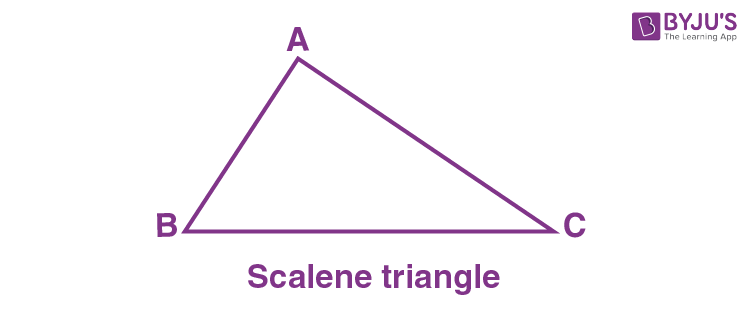In the above triangle ABC, the sides AB, BC and AC are not equal to each other.

AB ≠ BC ≠ AC

Also, the angles ∠A, ∠B and ∠C, are unequal in measure.

∠A ≠ ∠B ≠ ∠C

Thus, we can use the angle sum property to find each interior angle.

### Right Triangle

A right triangle is also an irregular polygon because it has an angle always equal to 90 degrees, and also the side opposite to the right angle is the longest side. Hence, neither the three sides are equal nor the three angles are equal in measure.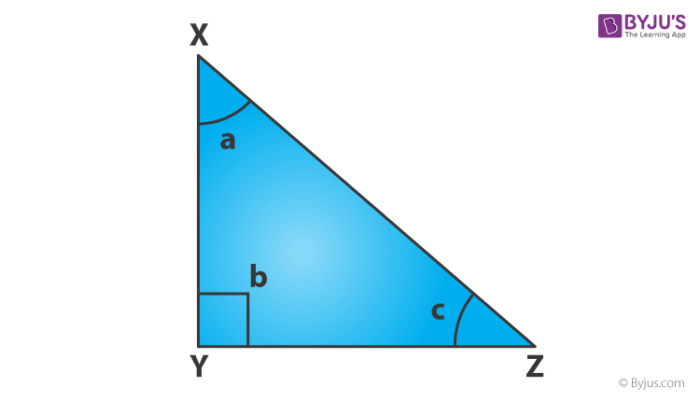In the above figure, the sides XY, YZ and XZ are not equal to each other.

XY = YZ = XZ, where XZ > XY & XZ > YZ

And,

∠a ≠ ∠b ≠ ∠c, where ∠b = 90°

### Isosceles Triangle

An isosceles triangle is a triangle that has any two sides equal in length (out of three) and the angles opposite to equal sides are equal in measure. Therefore, it is also an irregular polygon.

Also check: Isosceles Triangle

### Rectangle

A rectangle is also an irregular shape that has only its opposite sides equal in length. But all the angles are equal to 90 degrees. See the figure below.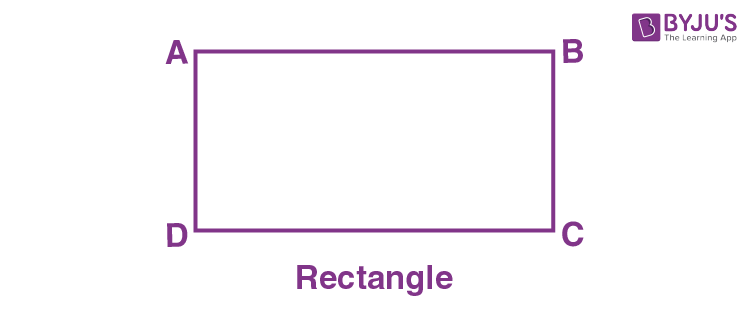In the given rectangle ABCD, the sides AB & CD are equal and BC and AD are equal.

AB = CD & BC = AD

And

∠A = ∠B = ∠C = ∠D = 90 degrees

But,

BC ≠ AB or CD

Hence, the rectangle is an irregular polygon.

### Irregular Pentagon

An irregular pentagon is a shape that has five unequal sides. It is possible to have two or three sides of an irregular pentagon equal in length but not all the sides are equal to each other. Learn the difference between regular and irregular pentagons.

### Irregular Hexagon

A hexagon is called irregular when its six sides are not equal in length to each other. Also, the measurements of each interior angle are not equal.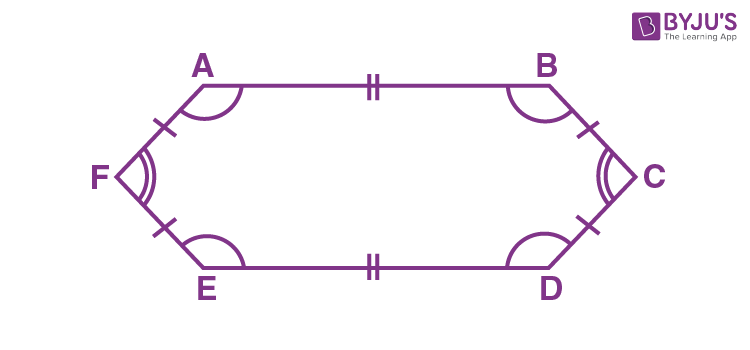By the above figure of hexagon ABCDEF, the opposite sides are equal but not all the sides AB, BC, CD, DE, EF and AF are equal to each other. Since the sides are not equal thus, the angles will also not be equal to each other. Therefore, an irregular hexagon is an irregular polygon.

 Fact: The polygon that is not regular is an irregular polygon.

## Frequently Asked Questions on Regular and Irregular Polygons

Q1

### What are regular polygons? Give an example.

A regular polygon is a polygon with sides equal in length and angles equal in measure. For example, a square has all four sides equal and all four angles equal to 90 degrees. Hence, it is a regular polygon.

Q2

### What are irregular polygons? Give an example.

A polygon is irregular when its sides are not equal to each other and the measure of angles may also be unequal. For example, a rectangle is a four-sided polygon that has only opposite sides equal but the angles are all equal in measure. Hence, it is an irregular polygon.

Q3

### What is the difference between regular and irregular polygons?

Regular polygons have all sides equal in length but an irregular polygon does not have all sides equal.

Q4

### What do we call a four-sided polygon?

A four-sided polygon is called a quadrilateral, whose sum of all four interior angles is equal to 360 degrees.

Q5

### Is the rhombus a regular polygon?

No, the rhombus is not a regular polygon. However, when the four angles of the rhombus are equal, it will be a regular polygon.